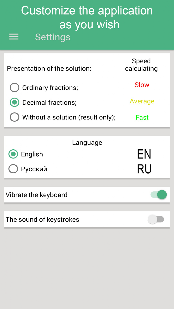Solution of systems of linear equations by the Jordan-Gauss method;

-Solution of systems of linear equations by the Cramer method;
-Solution of systems of linear equations by the Gauss method;
-Finding the determinant of the matrix;
-Calculation of the transpose matrix;
-Matrix multiplication by number;
-Erection of a matrix in degree;
-Finding the inverse matrix;
-Matrix rank calculation;
-Matrix multiplication;
-Matrix subtraction;
All solutions are detailed, step by step

What’s New

• Added the ability to save solutions and choose the interface language

Screenshots

•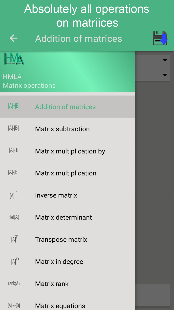•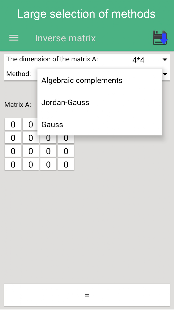••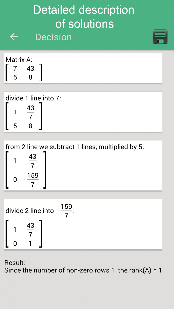•••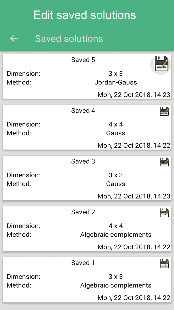•